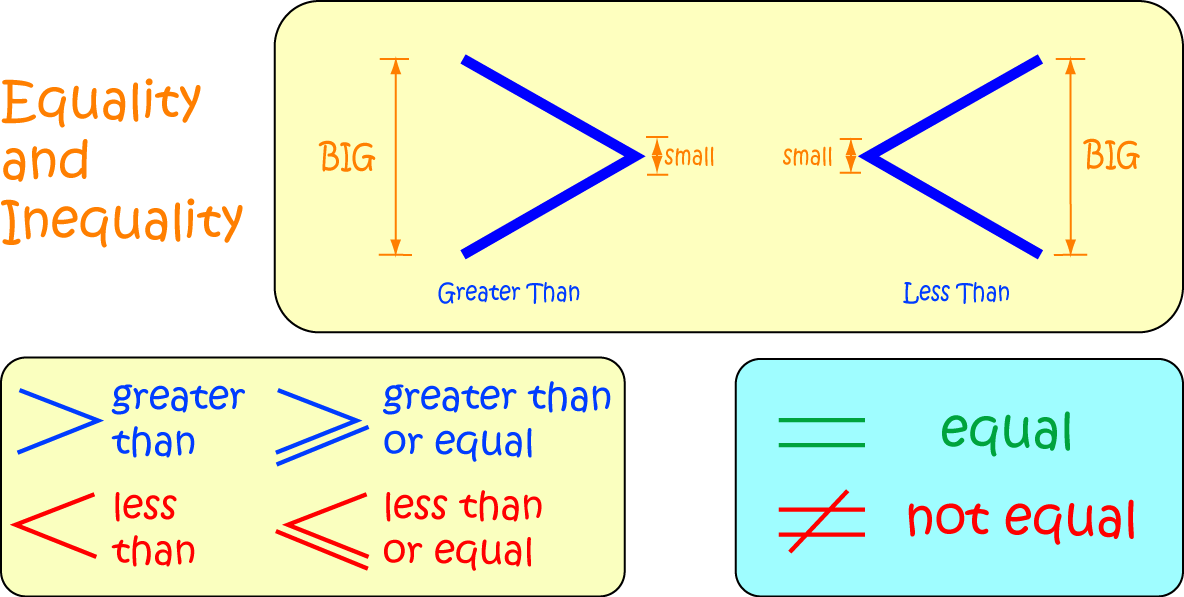# Definition of InequalityAn inequality is an expression that tells you that two values are not equal to each other.

There are a number of different symbols that tell you that two numbers are unequal, and which may describe the ways in which they differ from each other.

For example,

1. $x \neq y$ simply tells us that $x$ is not equal to $y$
2. $x 3. \(x > y$ tells us that $x$ is greater than $y$
4. $x \leq y$ tells us that $x$ is less than or equal to $y$
5. $x \geq y$ tells us that $x$ is greater than or equal to $y$

The symbols in 2. and 3. above are referred to as strict inequalities because they don't allow the two numbers to be equal.

### Description

The aim of this dictionary is to provide definitions to common mathematical terms. Students learn a new math skill every week at school, sometimes just before they start a new skill, if they want to look at what a specific term means, this is where this dictionary will become handy and a go-to guide for a student.

### Audience

Year 1 to Year 12 students

### Learning Objectives

Learn common math terms starting with letter I

Author: Subject Coach
You must be logged in as Student to ask a Question.